• FAQ
• Contact/ Homework Answers / Accounting / 41.The use of activity-based costing information to support the decision-making
Not my Question
Flag Content

# Question

41.The use of activity-based costing information to support the decision-making process is known as:

A. value chain analysis.

B. cost distortion analysis.

C. activity-based management.

D. cost-based management.

Essay Questions

42.For each of the following costs, check the columns that most likely apply.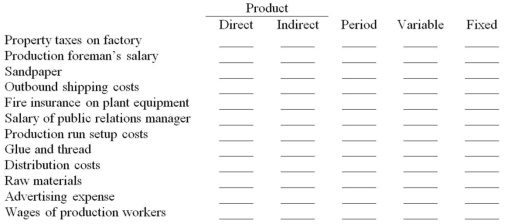43.Webster World Products uses the following account titles: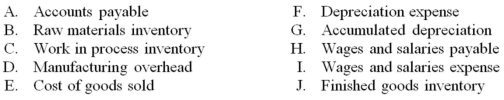For each transaction described below, indicate which accounts would be debited and credited under a job order cost system: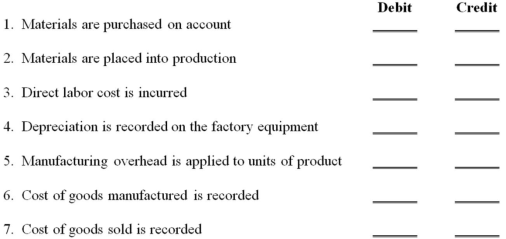44.Erca, Inc. produces automobile bumpers. Overhead is applied on the basis of machine hours required for cutting and fabricating. A predetermined overhead application rate of \$15.00 per machine hour was established for 2013.

(a.) If 9,000 machine hours were expected to be used during 2013, how much overhead was expected to be incurred?
(b.) Actual overhead incurred during 2013 totaled \$135,000, and 9,200 machine hours were used during 2013. Calculate the amount of over-or underapplied overhead for 2013.
(c.) Explain the accounting necessary for the over- or underapplied overhead for the year.

45.Vincent Custom Graphics estimates the following for 2013: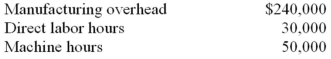During 2013 Vincent incurs the following costs and activity: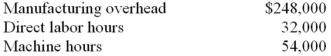Vincent uses direct labor hours to calculate the predetermined overhead rate for the year.

(a.) Calculate the predetermined overhead rate for 2013.
(b.) Calculate the applied overhead for 2013.
(c.) Calculate the amount of overapplied or underapplied overhead for 2013.

46.For each of the following costs, check the columns that most likely apply.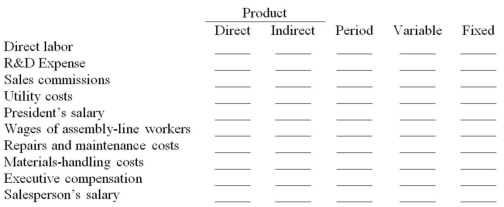47.George's Garage incurred the following costs during May: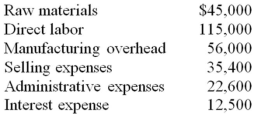During the month, 6,000 units of product were manufactured and 5,500 units of product were sold. On May 1, George's carried no inventories.

(a.) Calculate the cost of goods manufactured during May and the average cost per unit of product manufactured.
(b.) Calculate the cost of goods sold during May.
(c.) Where in the financial statements will the difference between cost of goods manufactured and cost of goods sold be classified?

48.Erber, Inc. produces men's neckties and dress socks. Manufacturing overhead is assigned to production using an application rate based on direct labor hours.

(a.) For 2013, the company's cost accountant estimated that total overhead costs incurred would be \$184,500, and that a total of 24,600 direct labor hours would be worked. Calculate the amount of overhead to be applied for each direct labor hour worked on a production run.
(b.) A production run of 500 neckties required raw materials that cost \$3,120, and 140 direct labor hours at a cost of \$8.00 per hour. Calculate the cost of each necktie produced.
(c.) At the end of February 2013, 420 neckties made in the above production run had been sold, and the rest were in ending inventory. Calculate the cost of the neckties sold that would have been reported in the income statement and the cost included in the February 28, 2013, finished goods inventory.

49.Partridge, Inc. incurred the following costs during March: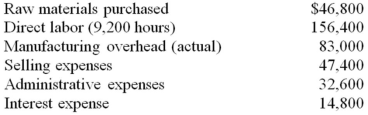Manufacturing overhead is applied on the basis of \$8.50 per direct labor hour. Assume that overapplied or underapplied overhead is transferred to cost of goods sold only at the end of the year. During the month, 3,500 units of product were manufactured and 3,400 units of product were sold. On March 1 and March 31, Partridge carried the following inventory balances: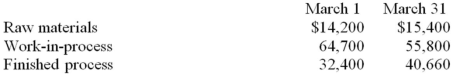(a.) Prepare a Statement of Cost of Goods Manufactured for the month of March, and calculate the average cost per unit produced.
(b.) Calculate the cost of goods sold during March.
(c.) Where in the financial statements will the difference between cost of goods manufactured and cost of goods sold be classified?

50.The following table summarizes the beginning and ending inventories of Ariel Co. for the month of October: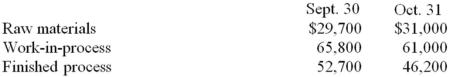Raw materials purchased during the month of October totaled \$112,300. Direct labor costs incurred totaled \$234,800 for the month. Actual and applied manufacturing overhead costs for October totaled \$145,100 and \$149,400, respectively.

(a) Calculate the cost of goods manufactured for October.
(b) Calculate the cost of goods sold for October (Ignore under/overapplied overhead).
(c) Given the fact that 25,000 units were produced, what is the cost per unit for October?

51.Great Bay Co. manufactures cordless telephones. During 2013, total costs associated with manufacturing 18,500 of the AB-2000 model (introduced this year) were as follows: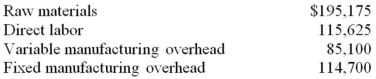(a.) Calculate the cost per phone under both direct (or variable) costing and absorption costing.
(b.) If 2,800 of these phones were in finished goods inventory at the end of 2013, by how much and in what direction (higher or lower) would 2013 operating income be different under direct (or variable) costing than under absorption costing?
(c.) Express the phone cost in a cost formula. What does this formula suggest the total cost of making an additional 1,600 phones would be?

52.Envision Toy Co. manufactures toy boats. During 2013, total costs incurred in making 27,000 toy boats included \$94,500 of fixed manufacturing overhead. The total absorption cost per toy boat was \$30.80.

(a.) Calculate the variable cost per toy boat.
(b.) The ending inventory of toy boats was 5,800 units higher at the end of 2013 than at the beginning of the year. By how much and in what direction (higher or lower) would cost of goods sold for 2013 be different under direct costing than under variable costing?
(c.) Express the toy boat cost in a cost formula. What does this formula suggest the total cost of making an additional 2,900 toy boats would be?

53.Fountain Company uses activity-based costing (ABC) for allocating manufacturing overhead costs to jobs and it has established the following cost drivers and rates: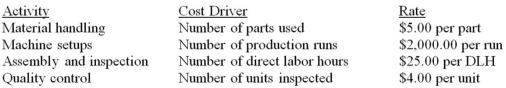During April, Job #7598 produced 2,500 units and required the following activity: 1,200 parts, 2 production runs, and 225 direct labor hours.

(a.) Calculate the amount of manufacturing overhead applied to Job #7598.
(b.) Explain the advantage of using the ABC approach.

54.Baja Industries has recently switched its method of applying manufacturing overhead from a single predetermined overhead rate based on direct labor hours to activity-based costing (ABC). Assume that the direct labor rate is \$18.00 per hour and that there were no beginning inventories. The following cost drivers and rates have been developed for allocating manufacturing overhead costs: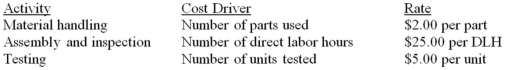The following production, costs, and activities occurred during the month of August: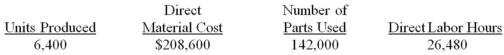(a.) Calculate the total manufacturing cost and the cost per unit for the month of August.
(b.) Assume instead that Baja Industries applies manufacturing overhead on the basis of \$40.00 per direct labor hours (rather than the ABC method). Calculate the total manufacturing overhead cost applied for the month of August.
(c.) Which method of applying overhead do you think provides better information for manufacturing managers?

13-1

## Solution 5 (1 Ratings )

Solved
Accounting 7 Months Ago 544 Views